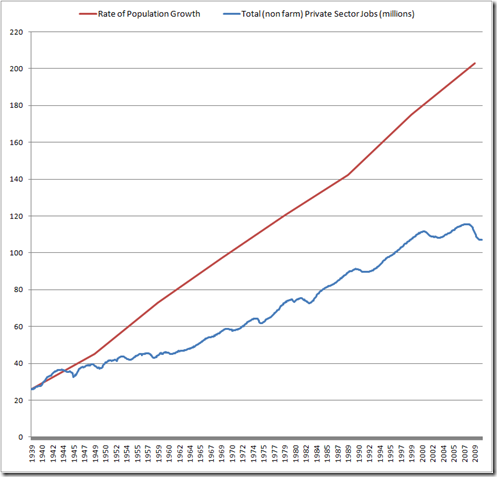2

# Jobs vs. Population (1939 – 2010)

This graph measures US Job growth for non-farm private sector jobs. For comparison, it includes the rate of US population growth.The population graph is based on the census data, which is only gathered every ten years – hence the smoothness. I was more interested in the rate of population growth than the actual total, so in order to get the lines next to each other for easier comparison, I subtracted 107 million from each census reading, which moved the population graph down next to the jobs graph without affecting the rate of growth.

Anyone could reproduce this graph by downloading data from the Bureau of Labor and Statistics web site into an Excel spreadsheet and then constructing this graph in Excel. However, I must give credit to the author of Econompic for giving me the idea in the first place. His graph of 1999 – 2010 data inspired me to construct this more comprehensive graph, which is what I was really looking for.

• Does red line represent "Population" or "Rate of Population Growth"?

• Probably red line is not "Rate of Population Growth" either.
What exactly does it mean?
Is it an increase in population since 1925?